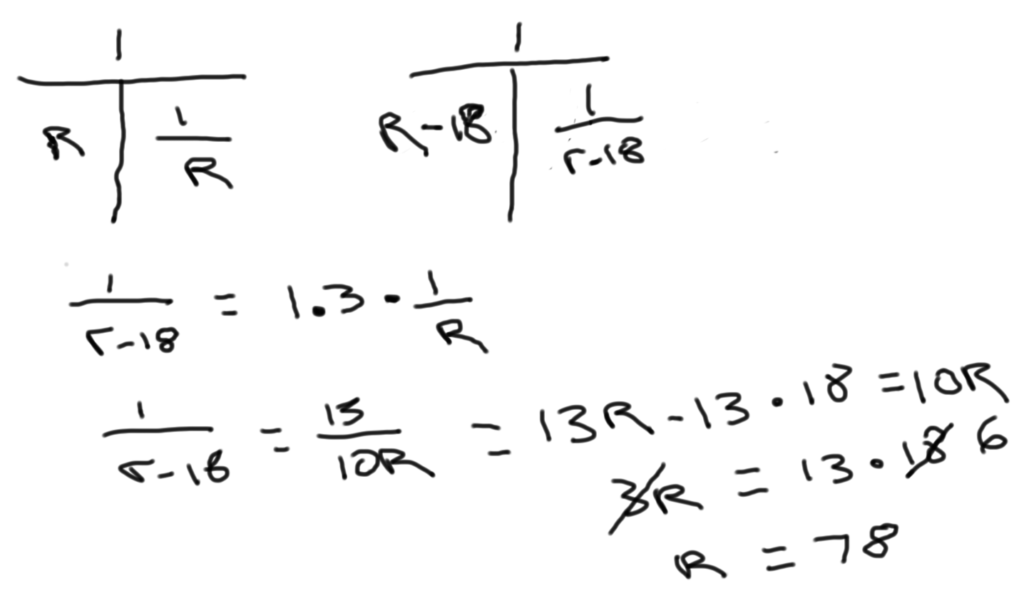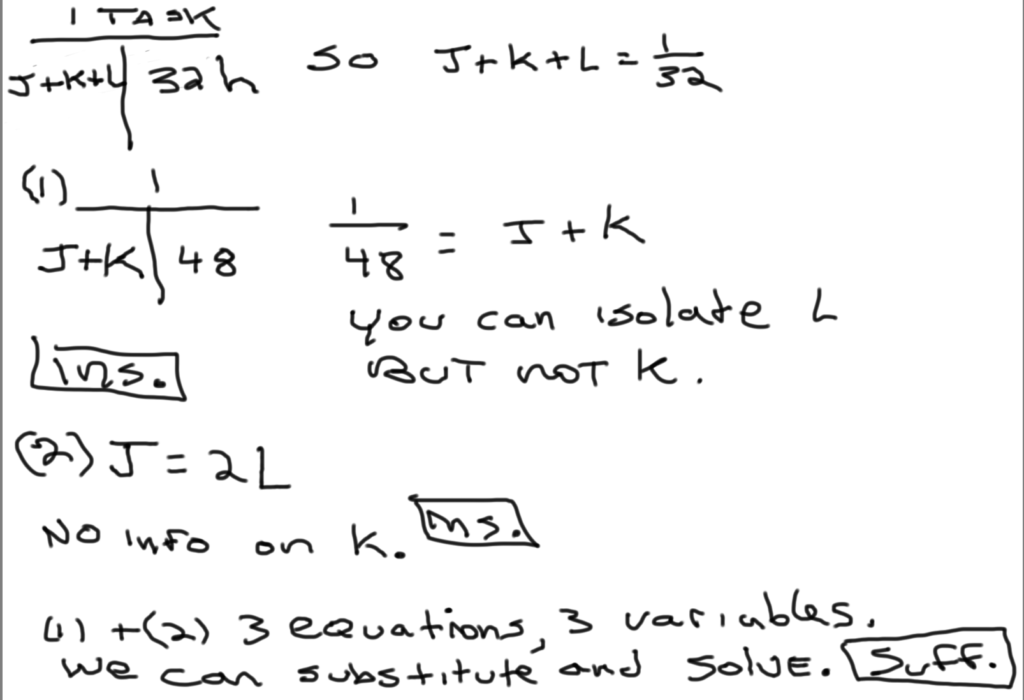Private GMAT Tutoring in NYC & Online

GMAT Question of the Day - PS Work/Rate - May 2nd, 2014

The express train would have taken 30% longer to get from Station K to Station M if it had been traveling 18 miles per hour slower. What was the express train's actual average speed, in miles per hour, from Station K to Station M?

A. 78

B. 82

C. 84

D. 85

E. 93

Here's an Official GMAT question on which we also use the T method to organize the information: A boat traveled upstream a distance of 90 miles at an average speed of (v-3) miles per hour and then traveled the same distance downstream at an average speed of (v+3) miles per hourDistance, Work and Rate - Average Rate 1

Distance, Work and Rate - Average Rate 2

For more GMAT distance, work, and rate practice visit GMAT Question of the Day.

GMAT Question of the Day - DS Rate/Work - April 29th 2014

Three machines J, K, and L working simultaneously but independently at their respective rates can complete a certain task in 32 hours. What proportion of the completed task did machine K complete?

(1) Machines J and K working simultaneously but independently at their respective rates can complete the task in 48 hours.

(2) Machine J can complete the task in half the time that Machine L can complete the task.Here are another bunch of cooperative work and rate questions to practice on:

Work and Rate 1

Work and Rate 2

Work and Rate 3

Work and Rate 4

Work and Rate 5

For more distance, work, and rate examples you can also check out our GMAT Question of the Day page

CONTACT

Atlantic GMAT

405 East 51st St.

NY, NY 10022

(347) 669-3545

info@AtlanticGMAT.com